# Sculpting and Debt Fees (Including Fees for DSRA L/C)

This page addresses how to incorporate debt fees and in particular fees on a DSRA letter of credit when you are sculpting debt repayments and structuring debt. Fees paid on debt, including fees paid on a letter of credit, may or may not be defined as part of debt service.  Fees that are included in debt service must be incorporated in developing sculpted repayment analysis. The discussion on this webpage demonstrates how the fundamental sculpting formulas that define debt at commercial operation as the present value of debt service can be adjusted to account for the debt fees by subtracting the present value of the fees from the fundamental debt sizing formulas. If the L/C fees or other fees such as political risk insurance are included as an operating expense, there are circular reference problems, but the debt fees do not have the NPV of fee adjustment that I discuss below. The amount of debt fees reduces the amount of debt that can be supported by cash flow — if you have the same cash flow and more fees, then the debt size from DSCR analysis will be reduced. As the size of the DSRA is affected by the fees and the fees depend on debt, a nasty circular reference arises.

There are a couple of key files where I put the financial formulas, modelling examples and the VBA code for cases where you run into circular references.  I have also made a file with some very simple examples that illustrate the formulas in cases where the interest rate is zero. Finally, there is a case where there are multiple debt issues with fees that are included in debt service. You can file these file on the google drive in the Project Finance Section under exercises and then Section D for the Sculpting course.  The final file is the circular reference resolution file attached to a model that deals with the DSRA as an L/C and includes provision for debt fees.

## Mechanics of Adjusting Debt Sizing for Fees with Debt Sizing from DSCR

In this paragraph I will try to demonstrate that you should include the fees in the sculpting equations, you should subtract the present value of fees that are included in the defined debt service when you compute the net present value of debt. To illustrate this, assume that the interest rate is zero and there are only fees on debt that compensate the lender (note that sometimes the fees that are included on a DSRA facility may not be included in debt service). If you pretend that the interest rate is zero and there are no fees, then the present value of the debt payment would be the sum of the repayments.  The sum of debt service without fees will produce too much debt.  Instead the total amount of the fees should be subtracted from the sum of debt service (again, I am still assuming that the interest rate is zero).

If there are either interest payments or fees, less debt service can support the same amount of cash flow to meet the DSCR constraint. This is illustrated in the very simple two period example below.  If there were no fees in the example, the debt would be 800 rather than 700 for the DSCR target of 1.5 on 600 per year of debt.  Here 600/1.5 is 400 and the total debt service over 2 periods is 800.  With fees there is less debt that can be covered by the 600 of cash flows.  The total debt service per period is still 400, but this consists also of fees.  Therefore the repayment — and also the debt balance — must be reduced.  Without an interest rate, the debt balance must be reduced by the amount of the fees. The cash flow is 600 and the allow debt service per year is then 600/1.5 = 400 which would pay off 800 of debt over two periods.  But with 50 of fees, the amount of the debt service for repayment is only 350 because 50 of fees are paid in the two periods. That is why the target debt service at the bottom of the simple example is less — 350 per year.  As the interest rate is zero, this represents the debt repayment and the total debt sums to 700.  The 700 could be derived by first dividing the cash flow by the DSCR to arrive at 400 debt service per year or 800.  Then, the PV of the fees (again at a zero interest rate) of 100 would yield total debt of 800 – 100 or 700.  The thing to remember is to subtract the PV of the fees.  But you should also remember to use the PV of cash flow without fees as the starting point.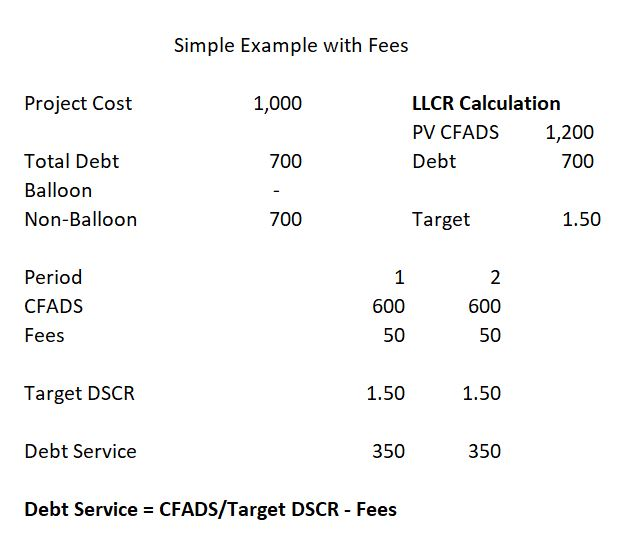When computing the NPV of debt service for sculpting in the presence of fees, you should reduce the PV of debt service for computing the fees. This is the NPV of debt service computed without fees must be lowered for the fees. Fees reduce the amount of debt service that can be supported by cash flow.  The fees reduce the amount of debt associated with CFADS compared to a situation without fees. Because the PV of debt service uses the debt interest rate without the effective rate that accounts for fees (which would be a higher interest rate), you can deduct the PV of fees at the debt interest rate and the debt schedule will work. To make the sculpting work you should also make the repayment lower by the fees as shown below:

DSCR = CFADS/(Repayment + Interest + Fees)

Repayment = CFADS/DSCR – Interest – Fees

Debt = NPV(Interest Rate, P&I – fees) = NPV(rate, Debt Service) – NPV(rate, Fees)

where the discount rate used in NPV is the interest rate (i.e. not adjusted for fees)

## Theory of Debt Fees after COD on an Letter of Credit that Replaces the Funded DSRA Account

It may be useful to review general some ideas of how debt fees fit into financial analysis. The fundamental point about fees is that debt fees are just like interest from the standpoint of a lender — money is earned by the lender on the basis of the amount of debt outstanding.  To evaluate the profitability of debt from the standpoint of a lender, you can compute the IRR earned. As with calculation of any IRR, the debt IRR is computed on the basis of cash flow coming out of your pocket compared to cash flow coming in.  The cash flow going out is the debt draws.  The cash flow coming in is the cash interest expense, plus the repayment and plus any debt fees before or after construction. The debt IRR which can also be called the all-in interest rate.

As debt fees that are paid after COD can be substituted for interest payments, it should be easy to see that debt fees should be included in debt service.  This means that debt fees should be included in the denominator of the DSCR.  You could even make an argument that the LLCR should be adjusted for debt fees as demonstrated in the formulas below:

With Fees: LLCR = NPV(CFADS)/NPV(Interest + Fees + Repayment) = NPV(CFADS)/(Debt + NPV(Fees)

## Mechanics of Sculpting with Fees when the Debt is Fixed

The bottom of the simple case below demonstrates the LLCR or the adjusted target DSCR can be adjusted. The PV of CFADS divided by the Debt plus the PV of Fees instead of just dividing the CFADS divided by the debt.  As with the case above, after you have computed the adjusted LLCR to compute the target debt service, the repayment is computed by subtracting the both fees and interest from debt service.

LLCR = PV CFADS/(Debt + PV Fees)

Repayment = Debt Service – Interest – Fees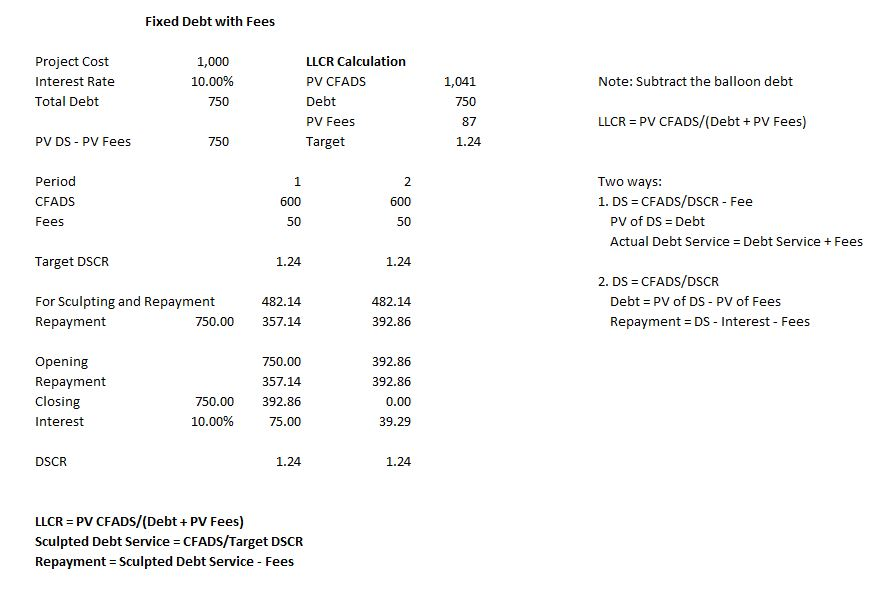## Analysis of Fees in Sculpting Exercise

A simple case with zero interest rate and a five percent interest rate is illustrated in the two screen shots below.  The first screen shot shows that you can just add up the required debt service, then subtract the sum of the fees and the target DSCR of 1.5 will be achieved.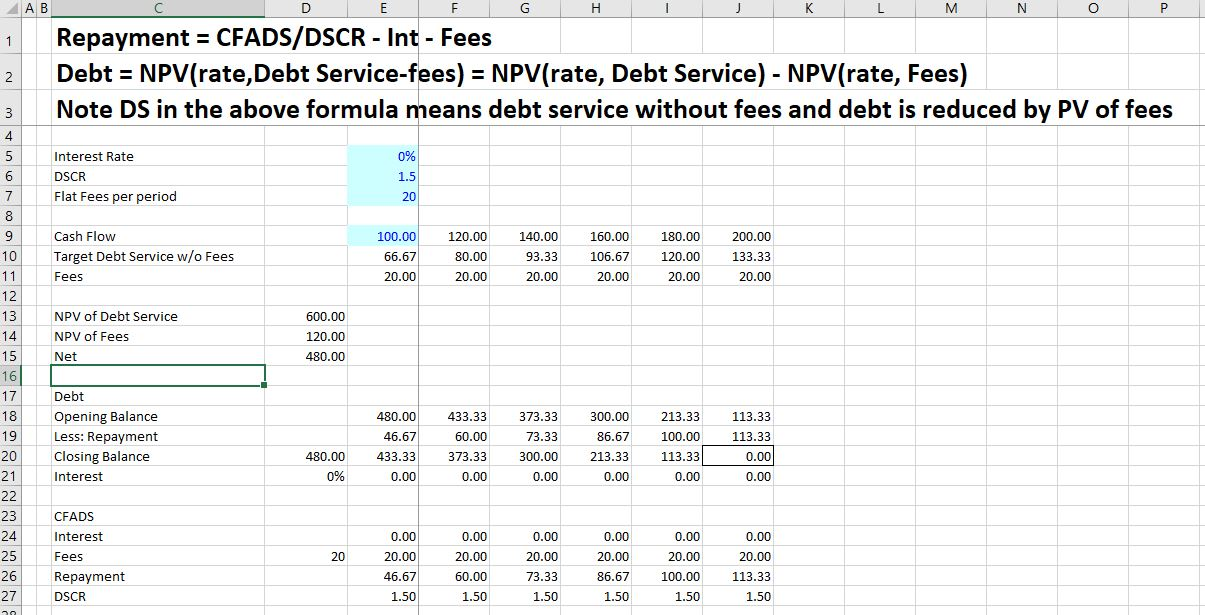The second screen shot demonstrates the case with a 10% interest rate.  There is lower debt in this case because of interest being paid, but the ideas are the same (the total debt amount falls from 480 to 332.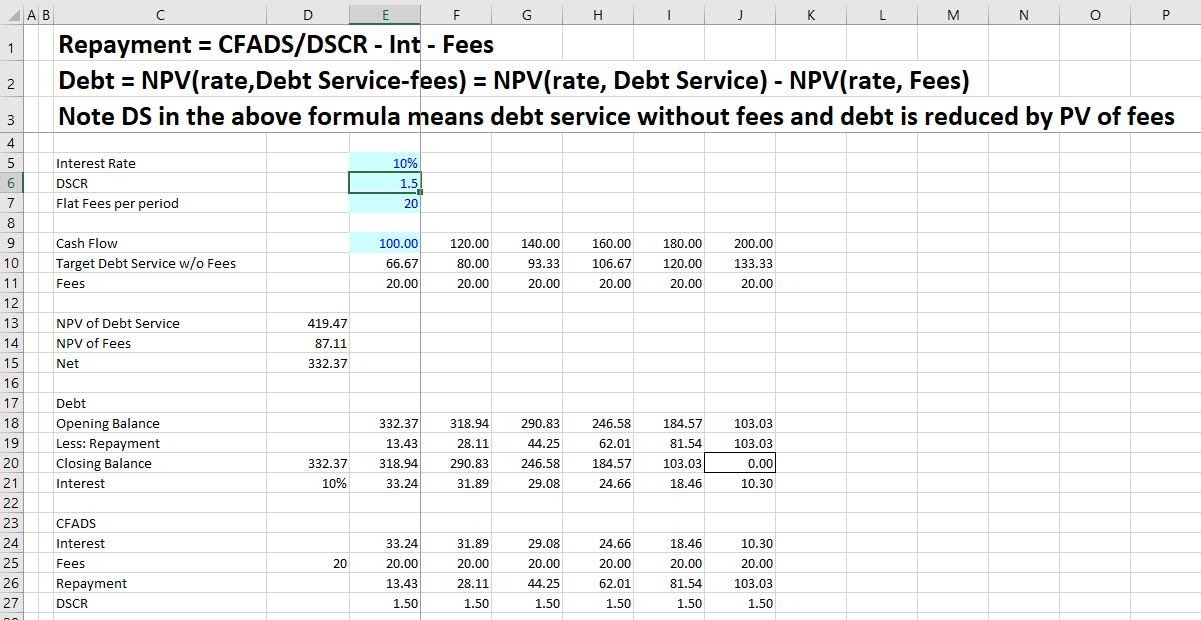Very often in sculpting, the debt is given and the repayments must be sculpted. When the debt is given, the fees affect the synthetic LLCR that is used to compute the debt service from the CFADS. In this case, the amount of repayment must be reduced because of the fees and the synthetic LLCR should be reduced. The sculpting analyses include calculation of the LLCR to evaluate whether the debt to capital constraint is driving the constraint. In this case the PV of CFADS is not the correct numerator for the analysis. Instead, the PV of the LC fees should be added to the denominator of the LLCR as follows:

LLCR = PV(CFADS)/(Debt + PV of LC Fees), where

Debt = Project Cost x Debt to Capital

A problem here is that the NPV of the debt depends on the fees, but the LC fees depend on the DSRA, which in turn depends on the size of the debt and the NPV. This is a clear circular reference. Note Debt Service in the above equation means debt service without fees and debt is reduced by PV of fees.

## Code with Adjustments for Debt Fees in Circular Reference Template

The code below is part of the circular reference template model. Even if you are a basic old copier and paster, the code can demonstrate the kinds of adjustments you should make in your project finance model.  The first excerpt shows the adjustments you should make in sizing the debt.

.

``` Select Case debt_sizing_option ' Define the amount of senior debt depending on the option choosen

Case 1: senior_debt = senior_fixed_total

Case 2: senior_debt = senior_percent_input * total_project_cost

Case 3: senior_debt = pv_debt_service - pv_fees + total_non_sculpt + DSRA_pv_adjustment

Case 4:
senior_debt_pct = senior_percent_input * total_project_cost

senior_debt_dscr = pv_debt_service_target_dscr - pv_fees + total_non_sculpt + DSRA_pv_adjustment ' Add other debt because PV debt service not includes

senior_debt = WorksheetFunction.Min(senior_debt_pct, senior_debt_dscr)

End Select```

.

The next excerpt shows how the LLCR computed for sculpting when the debt service constraint applies or multiple sculpting issues are implemented.

.

.

```'-------------------------------------------------------------------------------------------------------------------------------
' INITIAL DEBT SIZING SECTION: DETERMINE THE LLCR FOR USE IN SCULPTING WITH FIXED DEBT
'
' Determine what DSCR should be applied for sculpting
' Section for determining DSCR to use in sculpting when the debt balance is given
' The adjusted DSCR applied is used in computing the PV of debt service from CFADS
'-------------------------------------------------------------------------------------------------------------------------------

llcr = 0 ' This is the sculpting LLCR

LLCR_denominator = senior_debt + pv_fees - pv_non_sculpt

Select Case DSRA_Sculpting_Option

Case 2: LLCR_numerator = pv_cfads + pv_DSRA_flows
Case 3:
LLCR_denominator = senior_debt + pv_fees - pv_non_sculpt - pv_DSRA_flows
Case 4:
LLCR_numerator = pv_cfads + pv_DSRA_flows ' This is like case 2
LLCR_denominator = senior_debt + pv_fees - pv_non_sculpt

End Select

Select Case debt_sizing_option ' Take the LLCR or DSCR from the last iteration -- in the first iteration LLCR is 1

Case 1: ' This is the fixed debt case, for sculpting you need a DSCR

senior_debt = senior_debt_input

If LLCR_denominator > 0.1 And LLCR_numerator > 0.1 Then
llcr = LLCR_numerator / LLCR_denominator
Else
llcr = 1
End If

DSCR_Applied = llcr

Case 2: ' This is the case of debt to capital

senior_debt = senior_percent_input * total_project_cost

If LLCR_denominator <> 0 Then llcr = LLCR_numerator / LLCR_denominator

DSCR_Applied = llcr

Case 3: ' Where sculpting defines the debt

senior_debt = pv_debt_service - pv_fees + total_non_sculpt

DSCR_Applied = dscr_input

' If repayments are fixed, then must come up with debt size differently

Case 4: ' Optimise the repayment

If LLCR_denominator <> 0 Then llcr = LLCR_numerator / LLCR_denominator

DSCR_Applied = WorksheetFunction.Max(llcr, dscr_input)

DSCR_Applied = dscr_input

End Select```

.

.

## The Painful Issue of Fees and Withholding Tax with Multiple Debt Issues

The issue of fees and sculpting with multiple debt issues has been driving me completely crazy.  One of the problems is that the basis for allocating debt size between the different issues is the present value of the debt service before allocating the fees.  But the debt amount that drives that the repayment of each debt issue is affected by the fees. This means the amount of the debt for each issue must be adjusted for the amount of the fees.  The screenshots and the video below demonstrate the issue that has also been incorporated into the UDF. I have illustrated the problems and solutions with a simple example — no taxes and only two debt issues.  The example is illustrated in the screenshot below where there the two issues have different tenures, different interest rates and different fees.  The idea is to find the amount of debt that will lead to the target DSCR of 1.3 and also the 45%/55% target split between the two issues. The screenshot demonstrates that computed debt confirms to the target.  The excel file that you can download is attached to the button below.  In working through the issues, I hope you can see how the UDF concept works.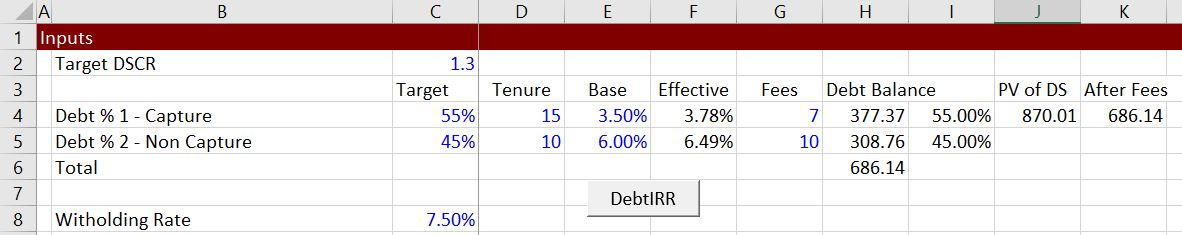### Step 1: Compute the PV of the Fees at Individual Effective Rates

The first step is to compute the present value of the fees at discount rates that are based on the interest rates of the separate debt issues.  This is demonstrated in the screenshot below.  Note that the present value is computed using the effective rate after the withholding tax.  The withholding tax that increases the effective rate is computed as the rate + rate x withholding tax/(1-withholding tax).  It is necessary to divide by 1-withholding tax becase the tax is compute on total interest including the withholding tax itself.  The total PV of fees is also computed because the total PV of fees is necessary for other calculations. The code in the UDF that confirms to the equiations for the PV of Fees is shown below the screenshot.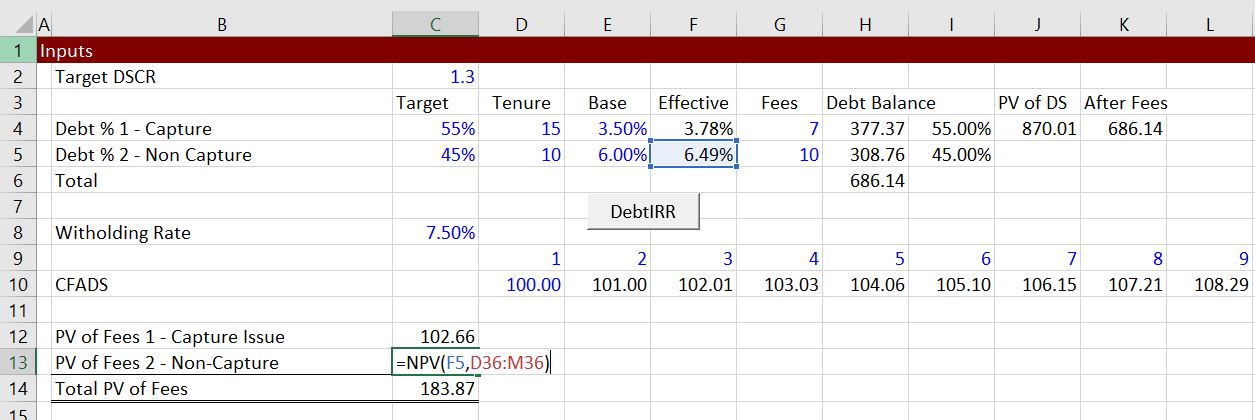```'-----------------------------------------------------------------------------------------------------------------------
' PV of CFADS BY ISSUE FOR ALL DEBT ISSUES INCLUDING THE CAPTURE DEBT
' First compute the CFADS of cash flow by issue which depends on the interest rate as well as the tenure
' Compute the PV of CFADS with Different debt tenures and different interest rates
' PV of CFADS is necessary for computing the LLCR by Issue
'-----------------------------------------------------------------------------------------------------------------------

pv_fees = 0

For j = 1 To total_debt_issues ' Loop through each issue

If sculpt_switch(j) = True And Debt_type_code(j) = 1 Then ' The whole next section is ignored if there is no sculpted issue

llcr_issue(j) = 5 ' Initialise LLCR in case there is a divide by zero problem
pv_cash_flow(j) = 0
int_index = 1
pv_fees_by_issue(j) = 0

'
' Compute the PV of CFADS by issue that depends on the tenure and the interest rate -- This Applies to All Issues
' The Individual Discount Rate is adjusted for witholding
'
' This applies to all issues -- the capture issue and other issues
' The calculation is made using the specific interest rate
'
For i = start_repayment To end_repayment ' end repayment is max tenure

If i <= debt_tenure(j) + start_repayment - 1 Then ' Loop Around Different Tenures
End If

after_tax_interest_rate = periodic_interest_rate(j, i) * (1 + effective_witholding_rate(i))
int_index = int_index * (1 + after_tax_interest_rate) ' Compound the interest rate

pv_cash_flow(j) = pv_cash_flow(j) + cfads_issue(i) / int_index ' Accumulate the PV of CFADS for LLCR
pv_fees_by_issue(j) = pv_fees_by_issue(j) + fees_by_issue(j, i) / int_index

Next i

pv_fees = pv_fees + pv_fees_by_issue(j)

```

### Part 2: Compute the Total Debt for Allocation Using the Overall Debt IRR

The total debt service is straightforward.  It is derived by the total debt service that has nothing to do with how the debt service is divided between fees and other items.  The PV is computed using a rate that includes the withholding taxes as the debt service includes the withholding fees.  But since the PV does not account for the fees, the PV of fees should be subtracted in computing the total debt that is subsequently used for allocating the debt between the issues.  Computation of the total debt is illustrated in the screenshot below, where the total debt is derived from the present value of total debt service. Equations that conform to these ideas are shown below the screenshot.  Note that debt computed from the aggregate debt service must be distinguished from other definitions of total debt (sorry this gets a confusing in the UDF.```'-----------------------------------------------------------------------------------------------------------------------------
' AGGREGATE SCULPT DEBT SERVICE SECTION - SCULPTING FOR SENIOR DEBT
'
' Now do the sculpting from CFADS for SENIOR Debt Issued During Construction
' Evaluate Sculpt the issue without re-financing before Re-financing

' First, adjust the timing to start with the period after the grace period and then continue to the debt tenor
'-----------------------------------------------------------------------------------------------------------------------------

' Aggregate_Debt_Service_With_Debt_IRR

' Set_Sculpting_Scalars_to_Zero_And_Compound_Interest_to_One

compound_interest = 1

start_repayment = start_operation + grace_period_sculpt
end_repayment = start_operation + senior_max_tenure - 1

For i = start_repayment To end_repayment

If Applied_Senior_DSCR_p(i) > 0 Then
Senior_sculpted_debt_service_p(i) = cfads_p(i) / Applied_Senior_DSCR_p(i) _
- senior_non_sculpt_debt_service_p(i) + balloon_repayment_p(i)

End If
'
' This is for case 4 where test alternatives
'
If Senior_dscr_input_array(i) <> 0 Then _
senior_debt_service_input_dscr = cfads_p(i) / Senior_dscr_input_array(i) _
- senior_non_sculpt_debt_service_p(i) + balloon_repayment_p(i) ' Amount for optional case

' Now compute the present value of Aggregate debt service and cfads for sculpting

interest_rate_p(i) = debt_IRR_senior ' This is from the debt IRR
after_tax_interest_rate = interest_rate_p(i)
compound_interest = compound_interest * (1 + after_tax_interest_rate)

'
' Compute the amount of the debt
'
pv_debt_service_senior_aggregate = _
pv_debt_service_senior_aggregate + Senior_sculpted_debt_service_p(i) / compound_interest ' Debt size from Applied DSCR

Next i ' End of repayment loop for aggregate sculpting```
```'------------------------------------------------------------------------------------------------------------------------------
'
' COMPUTE NON-CAPTURE Debt Issue LLCR and debt service
' Sculpting on these issues is defined by the issue-by-issue PV of CFADS/Debt Issue amount
' The debt issue amount is the total debt issue multiplied by the percent of the total
' senior_sculpt is the total amount of debt to allocate and the total non-capture debt service is accumulated
' The non-capture debt service calculation is the same no matter what method is used
'
'------------------------------------------------------------------------------------------------------------------------------

' Non_Capture_LLCR_And_Debt_Service

' Allocate the debt issue to compute the LLCR

'
' PV of debt service by issue is the amount of PV of debt service by issue and is not adjusted for fees
'

If j <> capture_j And percent_of_debt_applied(j) > 0 Then ' Only do this for non-capture issues

debt_balance_issue(j) = percent_of_debt_applied(j) * (pv_debt_service_senior_by_issue - pv_fees)```

### Step 3: Non-Capture Debt Amount, LLCR, Debt Service and Repayment

The### Step 4: Capture Debt Service, Debt Amount and Repayment

The capture debt service can be computed next.  This begins with computing the total debt service from the total and the capture debt service.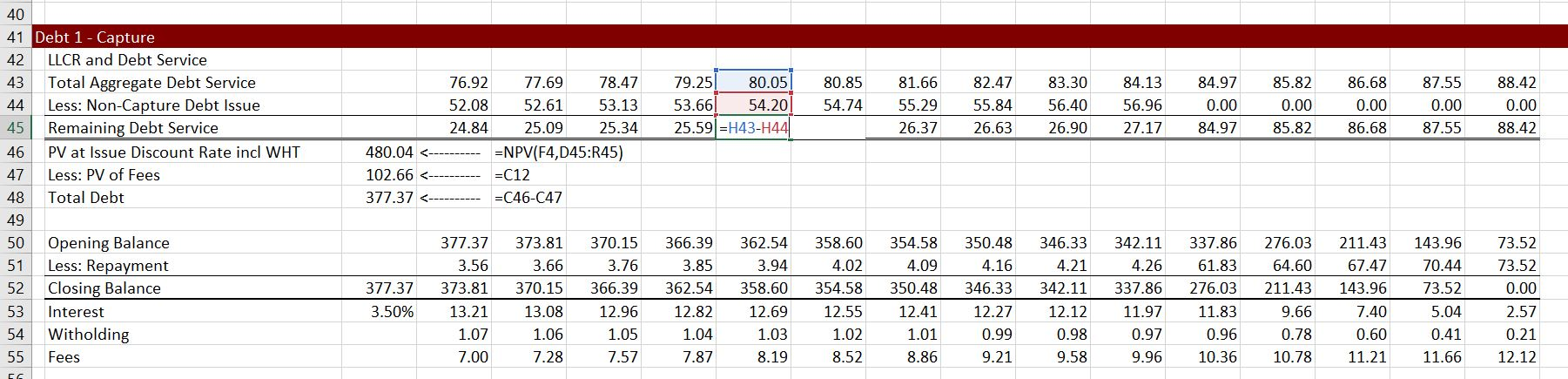### Step 5: Compute the Debt IRR from Total Debt Service and Computed Debt and PV of Fees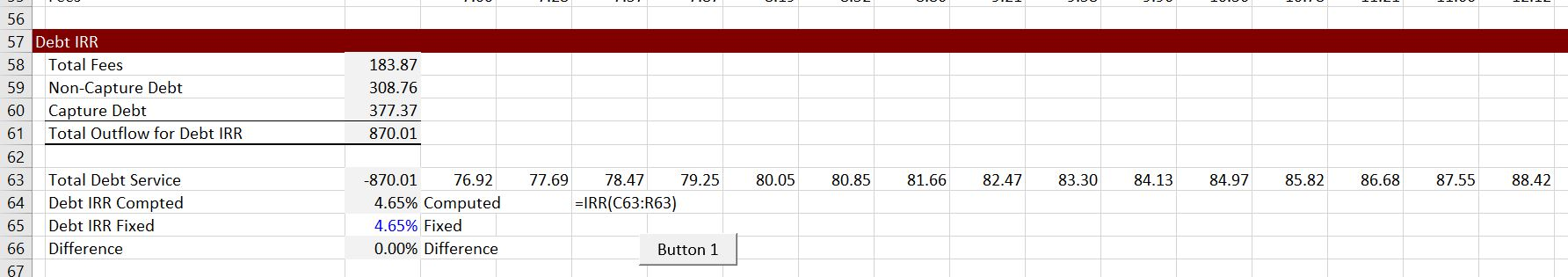## Further Information and Learning: Request Resource Library (Free), Find Details About In-Person Courses, Make Suggestions on Course Subjects and Locations

The reason I have worked on this website is so that you consider an in-person class which is by far the best way you can become a top project finance analyst.  If you click on the button below, you will be forwarded to a website that describes some of unique courses.

If you click on the right button you can quickly send an e-mail to edwardbodmer@gmail.com and request the resource library (no charge).  The google drives include more case studies, financial models, risk analysis files and other materials than are included on the website. I promise not to pester you if you do send me an e-mail.

I would really like to know what courses may be most interesting to you and where you would like the courses to be held. If you click on the left button below, I have a form that I will use to try and put together a class with a few people.

If you are a student, I would be honoured to come to your university or your business club and give you a hands-on guest lecture. If you click on the button on the left below, you can do me a big favor by giving me some information about your institution.